JEE  >  NCERT Solutions - Solutions (Part - 4)

# NCERT Solutions - Solutions (Part - 4) Notes | Study Additional Documents and Tests for JEE - JEE

## Document Description: NCERT Solutions - Solutions (Part - 4) for JEE 2022 is part of Additional Documents and Tests for JEE preparation. The notes and questions for NCERT Solutions - Solutions (Part - 4) have been prepared according to the JEE exam syllabus. Information about NCERT Solutions - Solutions (Part - 4) covers topics like and NCERT Solutions - Solutions (Part - 4) Example, for JEE 2022 Exam. Find important definitions, questions, notes, meanings, examples, exercises and tests below for NCERT Solutions - Solutions (Part - 4).

Introduction of NCERT Solutions - Solutions (Part - 4) in English is available as part of our Additional Documents and Tests for JEE for JEE & NCERT Solutions - Solutions (Part - 4) in Hindi for Additional Documents and Tests for JEE course. Download more important topics related with notes, lectures and mock test series for JEE Exam by signing up for free. JEE: NCERT Solutions - Solutions (Part - 4) Notes | Study Additional Documents and Tests for JEE - JEE
 1 Crore+ students have signed up on EduRev. Have you?

Q.35. Suggest the most important type of intermolecular attractive interaction in the following pairs.
(i) n-hexane and n-octane
(ii) I2 and CCl4
(iii) NaClO4 and water
(iv) methanol and acetone
(v) acetonitrile (CH3CN) and acetone (C3H6O).
Ans.
(i) Van der Waal’s forces of attraction.
(ii) Van der Waal’s forces of attraction.
(iii) Ion-dipole interaction.
(iv) Dipole-dipole interaction.
(v) Dipole-dipole interaction.

Q.36. Based on solute-solvent interactions, arrange the following in order of increasing solubility in n-octane and explain. Cyclohexane, KCl, CH3OH, CH3CN.
Ans. n-octane is a non-polar solvent. Therefore, the solubility of a non-polar solute is more than that of a polar solute in the n-octane.
The order of increasing polarity is:
Cyclohexane < CH3CN < CH3OH < KCl
Therefore, the order of increasing solubility is:
KCl < CH3OH < CH3CN < Cyclohexane

Q.37. Amongst the following compounds, identify which are insoluble, partially soluble and highly soluble in water?
(i) Phenol
(ii) Toluene
(iii) Formic acid
(iv) Ethylene glycol
(v) Chloroform
(vi) Pentanol.
Ans.
(i) Phenol (C6H5OH) has the polar group −OH and non-polar group −C6H5. Thus, phenol is partially soluble in water.
(ii) Toluene (C6H5−CH3) has no polar groups. Thus, toluene is insoluble in water.
(iii) Formic acid (HCOOH) has the polar group −OH and can form H-bond with water. Thus, formic acid is highly soluble in water.
(iv) Ethylene glycol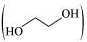has polar −OH group and can form H−bond. Thus, it is highly soluble in water.
(v) Chloroform is insoluble in water.
(vi) Pentanol (C5H11OH) has polar −OH group, but it also contains a very bulky non-polar −C5H11 group. Thus, pentanol is partially soluble in water.

Q.38. If the density of some lake water is 1.25 g mL−1 and contains 92 g of Na  ions per kg of water, calculate the molality of Na  ions in the lake.
Ans. Number of moles present in 92 g of Na+ ions = 92g / 23g mol-1 = 4 mol
Therefore, molality of Na + ions in the lake = 4 mol / 1 kg = 4 m

Q.39. Vapour pressure of pure acetone and chloroform at 328 K are 741.8 mm Hg and 632.8 mm Hg respectively. Assuming that they form ideal solution over the entire range of composition, plot ptotalpchloroform’ and pacetone as a function of xacetone. The experimental data observed for different compositions of mixture is.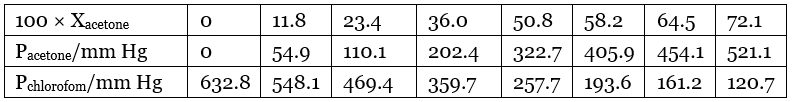Ans. From the question, we have the following data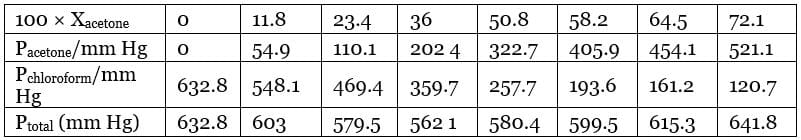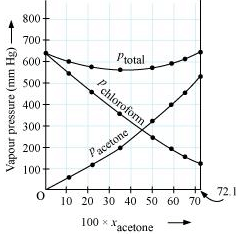It can be observed from the graph that the plot for the ptotal of the solution curves downwards. Therefore, the solution shows negative deviation from the ideal behaviour.

Q.40. Calculate the mass percentage of aspirin (C9H8O4) in acetonitrile (CH3CN) when 6.5 g of C9H8O4 is dissolved in 450 g of CH3CN.
Ans. 6.5 g of C9H8O4 is dissolved in 450 g of CH3CN.
Then, total mass of the solution = (6.5 + 450) g = 456.5 g
Therefore, mass percentage of C9H8O4 = (6.5/456.5) × 100% = 1.424%

Q.41. The air is a mixture of a number of gases. The major components are oxygen & nitrogen with approximate proportion of 20% is to 79% by volume at 298 K. The water is in equilibrium with air at a pressure of 10 atm. At 298 K if the Henry’s law constants for oxygen and nitrogen are 3.30 × 107 mm & 6.51 × 107 mm respectively, calculate the composition of these gases in water.
Ans. Percentage of oxygen (O2) in air = 20 %
Percentage of nitrogen (N2) in air = 79%
Also, it is given that water is in equilibrium with air at a total pressure of 10 atm,
that is, (10 × 760) mm Hg = 7600 mm Hg
Therefore,
Partial pressure of oxygen,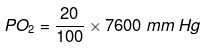= 1520 mm Hg
Partial pressure of nitrogen,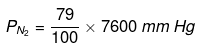= 6004 mmHg
Now, according to Henry’s law:
= KH.x
For oxygen: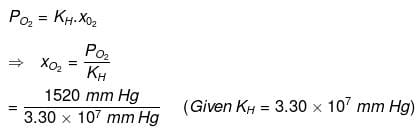= 4.61 x 10-5
For nitrogen: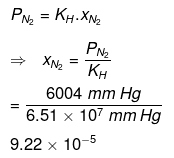Hence, the mole fractions of oxygen and nitrogen in water are 4.61 × 10−5 and 9.22 × 10−5 respectively.

Q.42. Benzene and toluene form ideal solution over the entire range of composition.The vapour pressure of pure benzene and naphthalene at 300 K are 50.71 mm Hg and 32.06 mm Hg respectively. Calculate the mole fraction of benzene in vapour phase if 80 g of benzene is mixed with 100 g of toluene.
Ans. Molar mass of benzene (C6H6) = 6 × 12 + 6 × 1 = 78 g mol-1
Molar mass of toluene (C6H5CH3) = 7 × 12 + 1 × 8 = 98 g mol-1
Now, no. of moles present in 80 g of benzene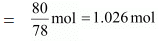And, no. of moles present in 100 g of toluene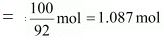∴ Mole fraction of benzene,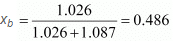And, mole fraction of toluene, x= 1 - 0.486 = 0.514
It is given that vapour pressure of pure benzene, P°b= 50.71 mm Hg
And, vapour pressure of pure toluene, P°t = 32.06 m Hg
Therefore, partial vapour pressure of benzene, p= xb × p°t
= 0.486 × 50.71
= 24.645 mm Hg
Hence, mole fraction of benzene in vapour phase is given by: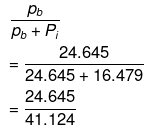= 0.599
= 0.6

Q.43. The depression in freezing point of water observed for the same amount of acetic acid, trichloroacetic acid and trifluoroacetic acid increases in the order given above. Explain briefly.
Ans.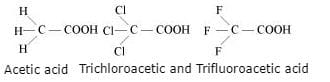Among H, Cl, and F, H is least electronegative while F is most electronegative. Then, F can withdraw electrons towards itself more than Cl and H. Thus, trifluoroacetic acid can easily lose H ions i.e., trifluoroacetic acid ionizes to the largest extent. Now, the more ions produced, the greater is the depression of the freezing point. Hence, the depression in the freezing point increases in the order:
Acetic acid < trichloroacetic acid < trifluoroacetic acid

Q.44. Calculate the depression in the freezing point of water when 10 g of CH3CH2CHClCOOH is added to 250 g of water. Ka = 1.4 × 10−3, K= 1.86 K kg mol−1.
Ans. Molar mass of CH3CH2CHClCOOH = 15 + 14 + 13 + 35.5 + 12 + 16 + 16 + 1 = 122.5 g mol-1
∴ No. of moles present in 10 g of CH3CH2CHClCOOH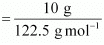= 0.0816mol
It is given that 10 g of CH3CH2CHClCOOH is added to 250 g of water.
∴ Molality of the solution,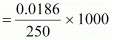= 0.3264 mol kg-1
Let α be the degree of dissociation of CH3CH2CHClCOOH undergoes dissociation according to the following equation: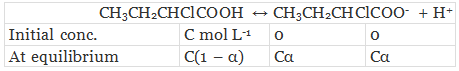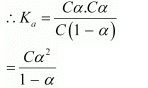Since α is very small with respect to 1, 1 − α ≈ 1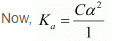⇒ Ka = Cα2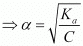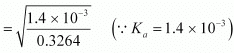= 0.0655
Again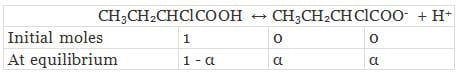Total moles of equilibrium = 1 − α + α + α
= 1 + α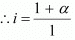Hence, the depression in the freezing point of water is given as:
ΔT= i.Kfm
= 1.0655 × 1.86 K kg mol-1 × 0.3264 mol kg-1
= 0.65 K

The document NCERT Solutions - Solutions (Part - 4) Notes | Study Additional Documents and Tests for JEE - JEE is a part of the JEE Course Additional Documents and Tests for JEE.
All you need of JEE at this link: JEE

## Additional Documents and Tests for JEE

11 videos|159 docs|14 tests
 Use Code STAYHOME200 and get INR 200 additional OFF

## Additional Documents and Tests for JEE

11 videos|159 docs|14 tests

Track your progress, build streaks, highlight & save important lessons and more!

,

,

,

,

,

,

,

,

,

,

,

,

,

,

,

,

,

,

,

,

,

;